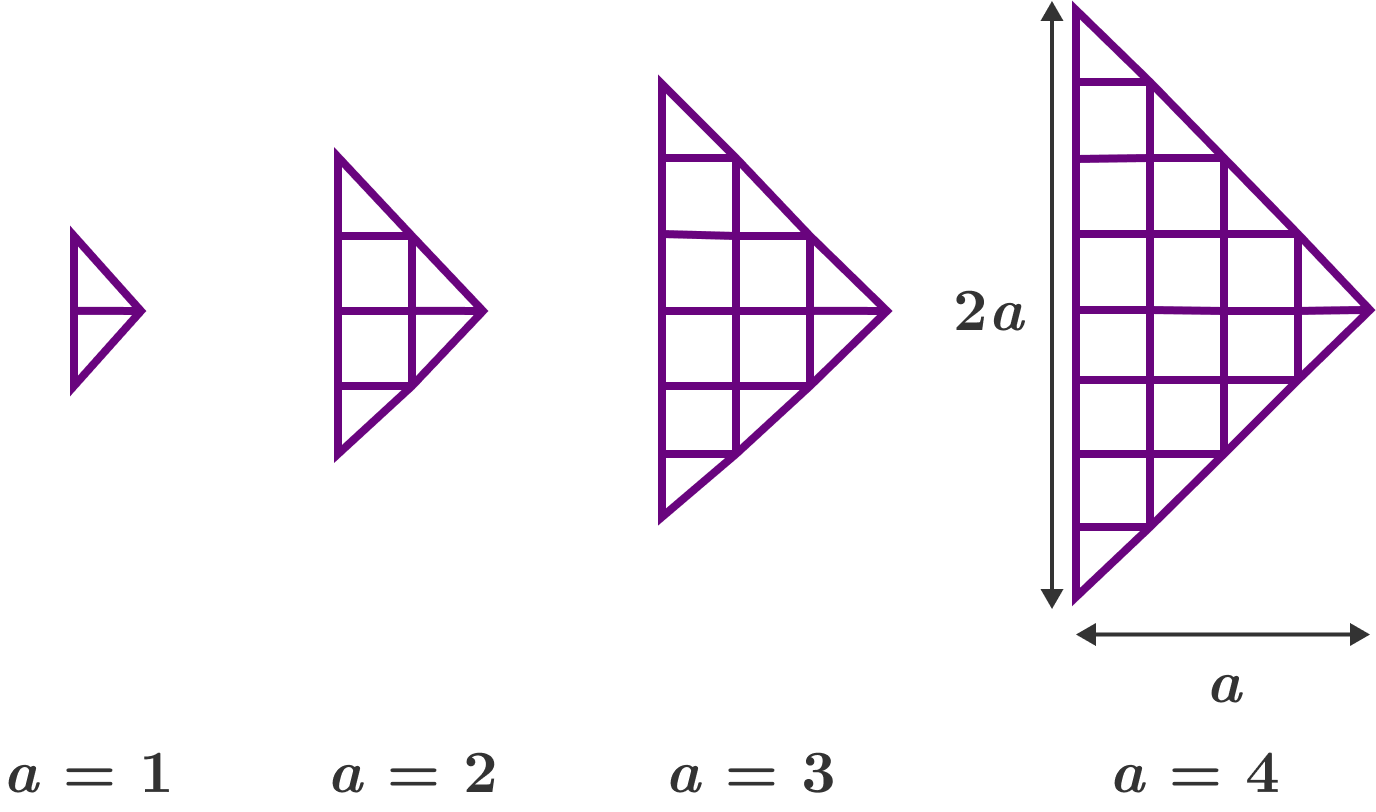# Mirroring the Slopes (Count 'em All 19!)

Probability Level 3

A right arrow grid with base length $2a$ is defined to be an $a \times a$ grid cut into 2 halves diagonally, and then put together as illustrated.

What is the total number of quadrilaterals in a right arrow grid with $a=30?$×